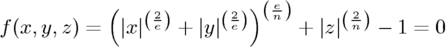POV-Ray : Documentation : 2.4.1.11 Superquadric EllipsoidPOV-Ray 3.6 Documentation Online ViewThe `superellipsoid` object creates a shape known as a superquadric ellipsoid object. It is an extension of the quadric ellipsoid. It can be used to create boxes and cylinders with round edges and other interesting shapes. Mathematically it is given by the equation:The values of `e` and `n`, called the east-west and north-south exponent, determine the shape of the superquadric ellipsoid. Both have to be greater than zero. The sphere is given by e = 1 and n = 1.

The syntax of the superquadric ellipsoid is:

```SUPERELLIPSOID:
superellipsoid
{
<Value_E, Value_N>
[OBJECT_MODIFIERS...]
}
```

The 2-D vector specifies the `e` and ` n` values in the equation above. The object sits at the origin and occupies a space about the size of a ` box{<-1,-1,-1>,<1,1,1>}`.

Two useful objects are the rounded box and the rounded cylinder. These are declared in the following way.

``` #declare Rounded_Box = superellipsoid { <Round, Round> }
#declare Rounded_Cylinder = superellipsoid { <1, Round> }
```

The roundedness value `Round` determines the roundedness of the edges and has to be greater than zero and smaller than one. The smaller you choose the values, the smaller and sharper the edges will get.

Very small values of `e` and `n` might cause problems with the root solver (the Sturmian root solver cannot be used).